Question

# Use implicit differentiation to find the equation of the tangent line at the given point. z?...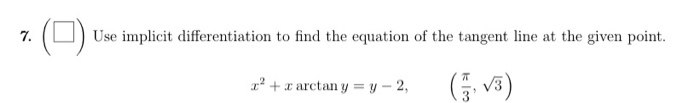Use implicit differentiation to find the equation of the tangent line at the given point. z? + x arctan y = y -2,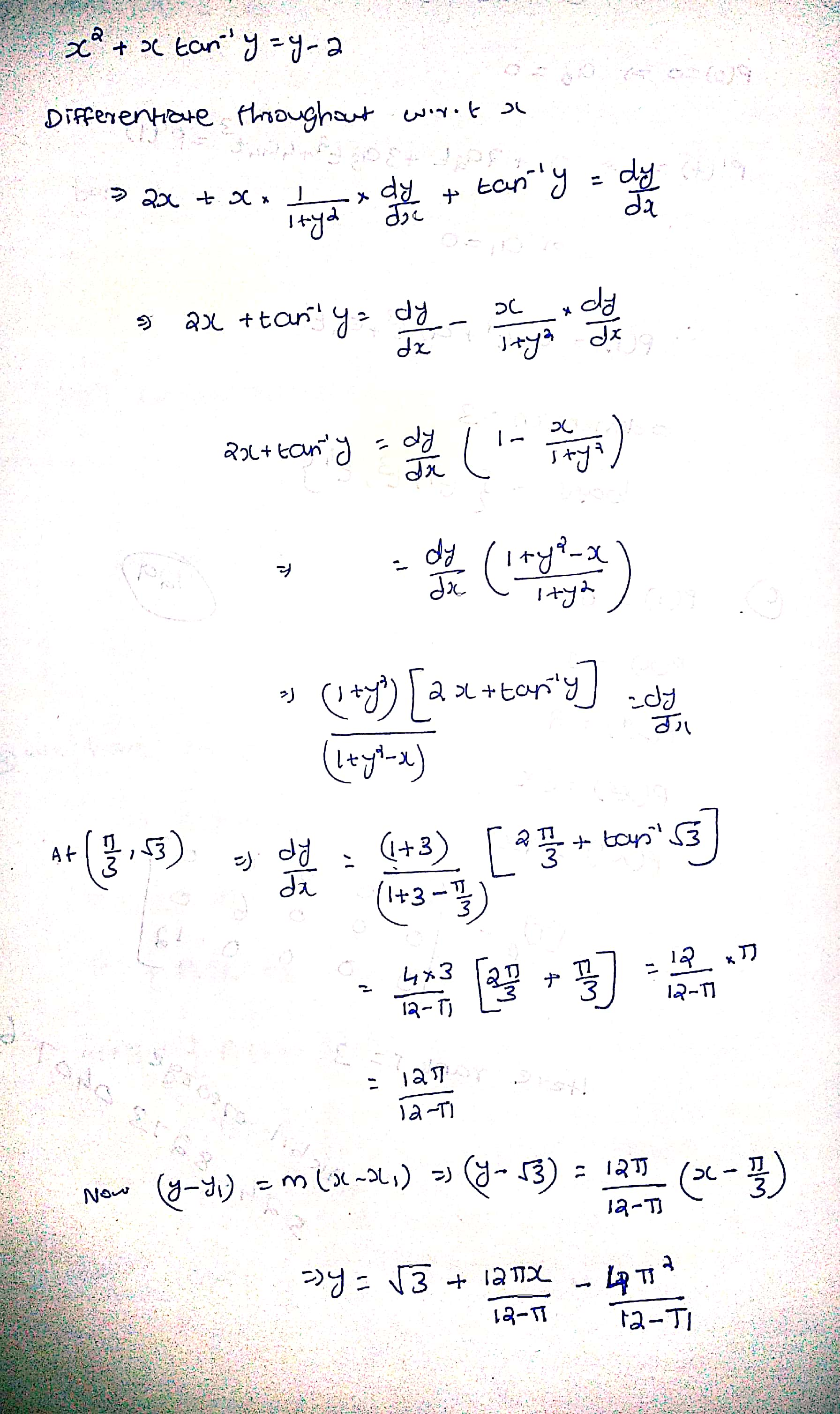#### Earn Coins

Coins can be redeemed for fabulous gifts.

Similar Homework Help Questions
• ### Use implicit differentiation to find an equation of the tangent line to the graph of the...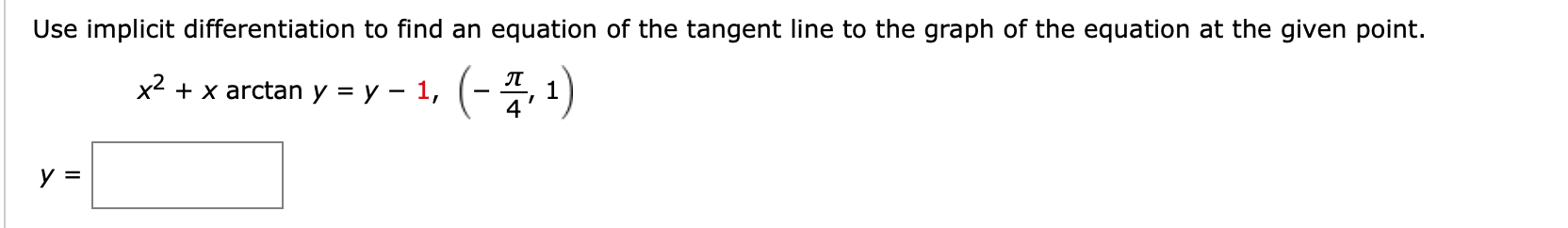Use implicit differentiation to find an equation of the tangent line to the graph of the equation at the given point. x2 + x arctan y = y -

• ### (1 point) Use implicit differentiation to find an equation of the tangent line to the curve...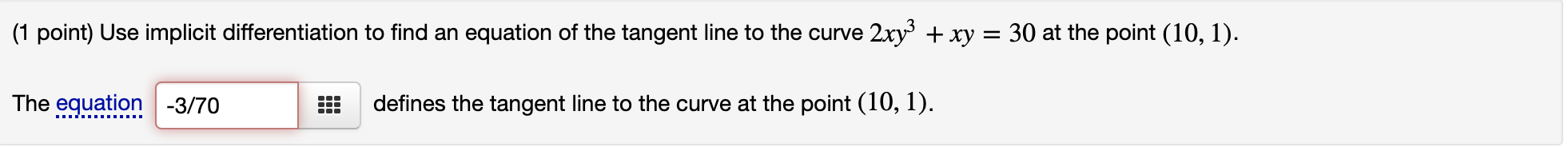(1 point) Use implicit differentiation to find an equation of the tangent line to the curve 2xy3+xy=302xy3+xy=30 at the point (10,1)(10,1). (1 point) Use implicit differentiation to find an equation of the tangent line to the curve 2xy3 + xy = 30 at the point (10, 1). The equation -3/70 defines the tangent line to the curve at the point (10, 1).

• ### Use implicit differentiation to find the equation of the tangent line to the curve xy3+xy=14 at the point (7,1)

Use implicit differentiation to find the equation of the tangent line to the curve xy3+xy=14 at the point (7,1) . The equation of this tangent line can be written in the form y=mx+bi dont seem to no how to find m or b

• ### Find the equation of the tangent line to the curve at the given point using implicit...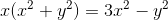Find the equation of the tangent line to the curve at the given point using implicit differentiation. Remember: equation of a line can be found by y-y1=m(x-x1) where m is the slope of the line and (x1,y1) is any point on the line. Curve: at (1,1)

• ### Use implicit differentiation to find the equation of the tangent line to the curve xy^3+2xy=9 at the point (31)

Use implicit differentiation to find the equation of the tangent line to the curve xy^3+2xy=9 at the point (31). The equation of this tangent line can be written in the form y=mx+b where m is

• ### Use implicit differentiation to find the equation of the tangent line to the curve xy^3+xy=5 at the point (4,1)

Use implicit differentiation to find the equation of the tangent line to the curve xy^3+xy=5 at the point (4,1) . The equation of this tangent line can be written in the form y = mx+b where m is:? and where b is:?

• ### 73. ♡ Use implicit differentiation to find an equation of the tangent line to the graph...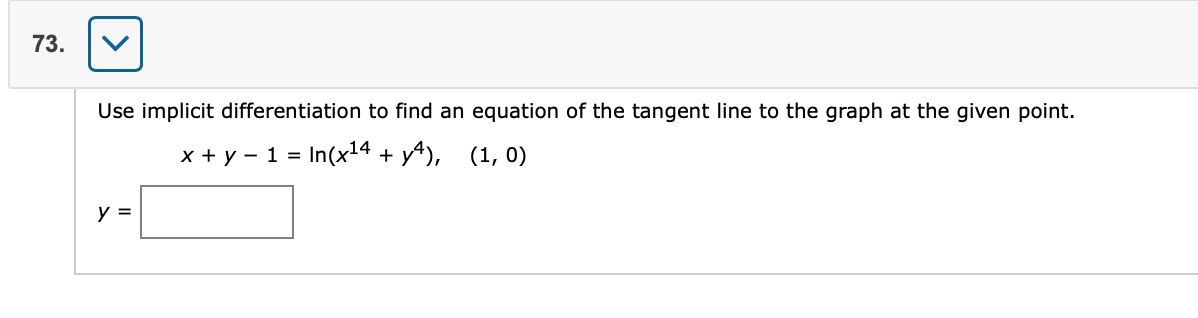73. ♡ Use implicit differentiation to find an equation of the tangent line to the graph at the given point. x + y - 1 = In(x14 + y4), (1, 0) Find the particular solution that satisfies the differential equation and the initial equations. f" 1) = 5, f(1) = 9, x > 0 y =

• ### (1 point) Use implicit differentiation to find the slope of the tangent line to the curve...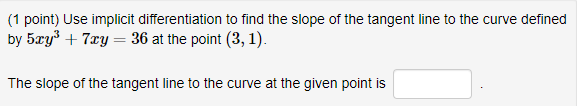(1 point) Use implicit differentiation to find the slope of the tangent line to the curve defined by 5xy + 7xy = 36 at the point (3,1). The slope of the tangent line to the curve at the given point is

• ### Use implicit differentiation to find an equation of the tangent line to the cardioid at the point (0,0.5) x^2+y^2=(2x^2+2y^2-x)^2 Y=

Use implicit differentiation to find an equation of the tangent line to the cardioid at the point (0,0.5)x^2+y^2=(2x^2+2y^2-x)^2Y=

• ### Find the slope of the tangent line to the implicit equation at the given point.

Find the slope of the tangent line to the implicit equation at the given point.F(X,Y)=x2 + xy + y2 -3 at (1,1)